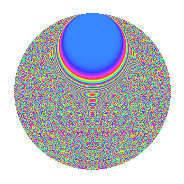# Properties

 Label 2541.2.bmLevel 2541 Weight 2 Character orbit bm Rep. character $$\chi_{2541}(233,\cdot)$$ Character field $$\Q(\zeta_{30})$$ Dimension 2176 Sturm bound 704

# Related objects

## Defining parameters

 Level: $$N$$ = $$2541 = 3 \cdot 7 \cdot 11^{2}$$ Weight: $$k$$ = $$2$$ Character orbit: $$[\chi]$$ = 2541.bm (of order $$30$$ and degree $$8$$) Character conductor: $$\operatorname{cond}(\chi)$$ = $$231$$ Character field: $$\Q(\zeta_{30})$$ Sturm bound: $$704$$

## Dimensions

The following table gives the dimensions of various subspaces of $$M_{2}(2541, [\chi])$$.

Total New Old
Modular forms 3008 2432 576
Cusp forms 2624 2176 448
Eisenstein series 384 256 128

## Trace form

 $$2176q + 3q^{3} + 262q^{4} + 20q^{6} + 20q^{7} + 9q^{9} + O(q^{10})$$ $$2176q + 3q^{3} + 262q^{4} + 20q^{6} + 20q^{7} + 9q^{9} - 24q^{12} + 40q^{13} - 12q^{15} + 206q^{16} + 5q^{18} + 10q^{19} + 25q^{24} - 202q^{25} - 6q^{27} - 10q^{28} + 35q^{30} - 256q^{34} + 28q^{36} + 6q^{37} + 45q^{39} + 120q^{40} + 6q^{42} - 16q^{45} + 50q^{46} - 94q^{48} + 48q^{49} + 45q^{51} + 10q^{52} - 60q^{57} - 28q^{58} + 87q^{60} + 50q^{61} - 60q^{63} - 224q^{64} + 32q^{67} - 78q^{69} - 68q^{70} - 55q^{72} + 70q^{73} - 49q^{75} - 340q^{78} + 90q^{79} - 31q^{81} - 10q^{82} - 125q^{84} - 160q^{85} - 30q^{90} + 192q^{91} + 30q^{93} + 210q^{94} - 135q^{96} + 40q^{97} + O(q^{100})$$

## Decomposition of $$S_{2}^{\mathrm{new}}(2541, [\chi])$$ into newform subspaces

The newforms in this space have not yet been added to the LMFDB.

## Decomposition of $$S_{2}^{\mathrm{old}}(2541, [\chi])$$ into lower level spaces

$$S_{2}^{\mathrm{old}}(2541, [\chi]) \cong$$ $$S_{2}^{\mathrm{new}}(231, [\chi])$$$$^{\oplus 2}$$

## Hecke Characteristic Polynomials

There are no characteristic polynomials of Hecke operators in the database